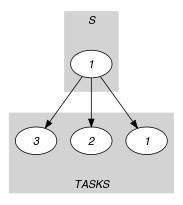## 5.353. sliding_time_window_from_start

Origin
Constraint

$\mathrm{𝚜𝚕𝚒𝚍𝚒𝚗𝚐}_\mathrm{𝚝𝚒𝚖𝚎}_\mathrm{𝚠𝚒𝚗𝚍𝚘𝚠}_\mathrm{𝚏𝚛𝚘𝚖}_\mathrm{𝚜𝚝𝚊𝚛𝚝}\left(\mathrm{𝚆𝙸𝙽𝙳𝙾𝚆}_\mathrm{𝚂𝙸𝚉𝙴},\mathrm{𝙻𝙸𝙼𝙸𝚃},\mathrm{𝚃𝙰𝚂𝙺𝚂},\mathrm{𝚂𝚃𝙰𝚁𝚃}\right)$

Arguments
 $\mathrm{𝚆𝙸𝙽𝙳𝙾𝚆}_\mathrm{𝚂𝙸𝚉𝙴}$ $\mathrm{𝚒𝚗𝚝}$ $\mathrm{𝙻𝙸𝙼𝙸𝚃}$ $\mathrm{𝚒𝚗𝚝}$ $\mathrm{𝚃𝙰𝚂𝙺𝚂}$ $\mathrm{𝚌𝚘𝚕𝚕𝚎𝚌𝚝𝚒𝚘𝚗}\left(\mathrm{𝚘𝚛𝚒𝚐𝚒𝚗}-\mathrm{𝚍𝚟𝚊𝚛},\mathrm{𝚍𝚞𝚛𝚊𝚝𝚒𝚘𝚗}-\mathrm{𝚍𝚟𝚊𝚛}\right)$ $\mathrm{𝚂𝚃𝙰𝚁𝚃}$ $\mathrm{𝚍𝚟𝚊𝚛}$
Restrictions
 $\mathrm{𝚆𝙸𝙽𝙳𝙾𝚆}_\mathrm{𝚂𝙸𝚉𝙴}>0$ $\mathrm{𝙻𝙸𝙼𝙸𝚃}\ge 0$ $\mathrm{𝚛𝚎𝚚𝚞𝚒𝚛𝚎𝚍}$$\left(\mathrm{𝚃𝙰𝚂𝙺𝚂},\left[\mathrm{𝚘𝚛𝚒𝚐𝚒𝚗},\mathrm{𝚍𝚞𝚛𝚊𝚝𝚒𝚘𝚗}\right]\right)$ $\mathrm{𝚃𝙰𝚂𝙺𝚂}.\mathrm{𝚍𝚞𝚛𝚊𝚝𝚒𝚘𝚗}\ge 0$
Purpose

The sum of the intersections of all the tasks of the $\mathrm{𝚃𝙰𝚂𝙺𝚂}$ collection with interval $\left[\mathrm{𝚂𝚃𝙰𝚁𝚃},\mathrm{𝚂𝚃𝙰𝚁𝚃}+\mathrm{𝚆𝙸𝙽𝙳𝙾𝚆}_\mathrm{𝚂𝙸𝚉𝙴}-1\right]$ is less than or equal to $\mathrm{𝙻𝙸𝙼𝙸𝚃}$.

Example
$\left(\begin{array}{c}9,6,〈\begin{array}{cc}\mathrm{𝚘𝚛𝚒𝚐𝚒𝚗}-10\hfill & \mathrm{𝚍𝚞𝚛𝚊𝚝𝚒𝚘𝚗}-3,\hfill \\ \mathrm{𝚘𝚛𝚒𝚐𝚒𝚗}-5\hfill & \mathrm{𝚍𝚞𝚛𝚊𝚝𝚒𝚘𝚗}-1,\hfill \\ \mathrm{𝚘𝚛𝚒𝚐𝚒𝚗}-6\hfill & \mathrm{𝚍𝚞𝚛𝚊𝚝𝚒𝚘𝚗}-2\hfill \end{array}〉,5\hfill \end{array}\right)$

The intersections of tasks $〈\mathrm{𝚒𝚍}-1\mathrm{𝚘𝚛𝚒𝚐𝚒𝚗}-10\mathrm{𝚍𝚞𝚛𝚊𝚝𝚒𝚘𝚗}-3〉$, $〈\mathrm{𝚒𝚍}-2\mathrm{𝚘𝚛𝚒𝚐𝚒𝚗}-5\mathrm{𝚍𝚞𝚛𝚊𝚝𝚒𝚘𝚗}-1〉$, and $〈\mathrm{𝚒𝚍}-3\mathrm{𝚘𝚛𝚒𝚐𝚒𝚗}-6\mathrm{𝚍𝚞𝚛𝚊𝚝𝚒𝚘𝚗}-2〉$ with interval $\left[\mathrm{𝚂𝚃𝙰𝚁𝚃},\mathrm{𝚂𝚃𝙰𝚁𝚃}+\mathrm{𝚆𝙸𝙽𝙳𝙾𝚆}_\mathrm{𝚂𝙸𝚉𝙴}-1\right]=\left[5,5+9-1\right]=\left[5,13\right]$ are respectively equal to 3, 1, and 2 (i.e., the three tasks of the $\mathrm{𝚃𝙰𝚂𝙺𝚂}$ collection are in fact included within interval $\left[5,13\right]$). Consequently, the $\mathrm{𝚜𝚕𝚒𝚍𝚒𝚗𝚐}_\mathrm{𝚝𝚒𝚖𝚎}_\mathrm{𝚠𝚒𝚗𝚍𝚘𝚠}_\mathrm{𝚏𝚛𝚘𝚖}_\mathrm{𝚜𝚝𝚊𝚛𝚝}$ constraint holds since the sum $3+1+2$ of these intersections does not exceed the value of its second argument $\mathrm{𝙻𝙸𝙼𝙸𝚃}=6$.

Typical
 $\mathrm{𝚆𝙸𝙽𝙳𝙾𝚆}_\mathrm{𝚂𝙸𝚉𝙴}>1$ $\mathrm{𝙻𝙸𝙼𝙸𝚃}>0$ $\mathrm{𝙻𝙸𝙼𝙸𝚃}<\mathrm{𝚆𝙸𝙽𝙳𝙾𝚆}_\mathrm{𝚂𝙸𝚉𝙴}$ $|\mathrm{𝚃𝙰𝚂𝙺𝚂}|>1$ $\mathrm{𝚃𝙰𝚂𝙺𝚂}.\mathrm{𝚍𝚞𝚛𝚊𝚝𝚒𝚘𝚗}>0$
Symmetries
• $\mathrm{𝚆𝙸𝙽𝙳𝙾𝚆}_\mathrm{𝚂𝙸𝚉𝙴}$ can be decreased.

• $\mathrm{𝙻𝙸𝙼𝙸𝚃}$ can be increased.

• Items of $\mathrm{𝚃𝙰𝚂𝙺𝚂}$ are permutable.

• $\mathrm{𝚃𝙰𝚂𝙺𝚂}.\mathrm{𝚍𝚞𝚛𝚊𝚝𝚒𝚘𝚗}$ can be decreased to any value $\ge 0$.

• One and the same constant can be added to $\mathrm{𝚂𝚃𝙰𝚁𝚃}$ as well as to the $\mathrm{𝚘𝚛𝚒𝚐𝚒𝚗}$ attribute of all items of $\mathrm{𝚃𝙰𝚂𝙺𝚂}$.

Arg. properties

Contractible wrt. $\mathrm{𝚃𝙰𝚂𝙺𝚂}$.

Reformulation

Similar to the reformulation of $\mathrm{𝚜𝚕𝚒𝚍𝚒𝚗𝚐}_\mathrm{𝚝𝚒𝚖𝚎}_\mathrm{𝚠𝚒𝚗𝚍𝚘𝚠}$.

Used in
Keywords
Derived Collection
$\mathrm{𝚌𝚘𝚕}\left(𝚂-\mathrm{𝚌𝚘𝚕𝚕𝚎𝚌𝚝𝚒𝚘𝚗}\left(\mathrm{𝚟𝚊𝚛}-\mathrm{𝚍𝚟𝚊𝚛}\right),\left[\mathrm{𝚒𝚝𝚎𝚖}\left(\mathrm{𝚟𝚊𝚛}-\mathrm{𝚂𝚃𝙰𝚁𝚃}\right)\right]\right)$
Arc input(s)

$𝚂$ $\mathrm{𝚃𝙰𝚂𝙺𝚂}$

Arc generator
$\mathrm{𝑃𝑅𝑂𝐷𝑈𝐶𝑇}$$↦\mathrm{𝚌𝚘𝚕𝚕𝚎𝚌𝚝𝚒𝚘𝚗}\left(𝚜,\mathrm{𝚝𝚊𝚜𝚔𝚜}\right)$

Arc arity
Arc constraint(s)
$\mathrm{𝚃𝚁𝚄𝙴}$
Graph property(ies)
$\mathrm{𝐒𝐔𝐌}_\mathrm{𝐖𝐄𝐈𝐆𝐇𝐓}_\mathrm{𝐀𝐑𝐂}$$\left(\begin{array}{c}\mathrm{𝚖𝚊𝚡}\left(\begin{array}{c}0,\begin{array}{c}\mathrm{𝚖𝚒𝚗}\left(\begin{array}{c}𝚜.\mathrm{𝚟𝚊𝚛}+\mathrm{𝚆𝙸𝙽𝙳𝙾𝚆}_\mathrm{𝚂𝙸𝚉𝙴},\hfill \\ \mathrm{𝚝𝚊𝚜𝚔𝚜}.\mathrm{𝚘𝚛𝚒𝚐𝚒𝚗}+\mathrm{𝚝𝚊𝚜𝚔𝚜}.\mathrm{𝚍𝚞𝚛𝚊𝚝𝚒𝚘𝚗}\hfill \end{array}\right)-\hfill \\ \mathrm{𝚖𝚊𝚡}\left(𝚜.\mathrm{𝚟𝚊𝚛},\mathrm{𝚝𝚊𝚜𝚔𝚜}.\mathrm{𝚘𝚛𝚒𝚐𝚒𝚗}\right)\hfill \end{array}\hfill \end{array}\right)\hfill \end{array}\right)\le \mathrm{𝙻𝙸𝙼𝙸𝚃}$

Graph model

Since we use the $\mathrm{𝚃𝚁𝚄𝙴}$ arc constraint the final and the initial graph are identical. The unique source of the final graph corresponds to the interval $\left[\mathrm{𝚂𝚃𝙰𝚁𝚃},\mathrm{𝚂𝚃𝙰𝚁𝚃}+\mathrm{𝚆𝙸𝙽𝙳𝙾𝚆}_\mathrm{𝚂𝙸𝚉𝙴}-1\right]$. Each sink of the final graph represents a given task of the $\mathrm{𝚃𝙰𝚂𝙺𝚂}$ collection. We associate to each arc the value given by the intersection of the task associated with one of the extremities of the arc with the time window $\left[\mathrm{𝚂𝚃𝙰𝚁𝚃},\mathrm{𝚂𝚃𝙰𝚁𝚃}+\mathrm{𝚆𝙸𝙽𝙳𝙾𝚆}_\mathrm{𝚂𝙸𝚉𝙴}-1\right]$. Finally, the graph property $\mathrm{𝐒𝐔𝐌}_\mathrm{𝐖𝐄𝐈𝐆𝐇𝐓}_\mathrm{𝐀𝐑𝐂}$ sums up all the valuations of the arcs and check that it does not exceed a given limit.

Parts (A) and (B) of Figure 5.353.1 respectively show the initial and final graph associated with the Example slot. To each arc of the final graph we associate the intersection of the corresponding sink task with interval $\left[\mathrm{𝚂𝚃𝙰𝚁𝚃},\mathrm{𝚂𝚃𝙰𝚁𝚃}+\mathrm{𝚆𝙸𝙽𝙳𝙾𝚆}_\mathrm{𝚂𝙸𝚉𝙴}-1\right]$. The constraint $\mathrm{𝚜𝚕𝚒𝚍𝚒𝚗𝚐}_\mathrm{𝚝𝚒𝚖𝚎}_\mathrm{𝚠𝚒𝚗𝚍𝚘𝚠}_\mathrm{𝚏𝚛𝚘𝚖}_\mathrm{𝚜𝚝𝚊𝚛𝚝}$ holds since the sum of the previous intersections does not exceed $\mathrm{𝙻𝙸𝙼𝙸𝚃}$.

##### Figure 5.353.1. Initial and final graph of the $\mathrm{𝚜𝚕𝚒𝚍𝚒𝚗𝚐}_\mathrm{𝚝𝚒𝚖𝚎}_\mathrm{𝚠𝚒𝚗𝚍𝚘𝚠}_\mathrm{𝚏𝚛𝚘𝚖}_\mathrm{𝚜𝚝𝚊𝚛𝚝}$ constraint(a) (b)